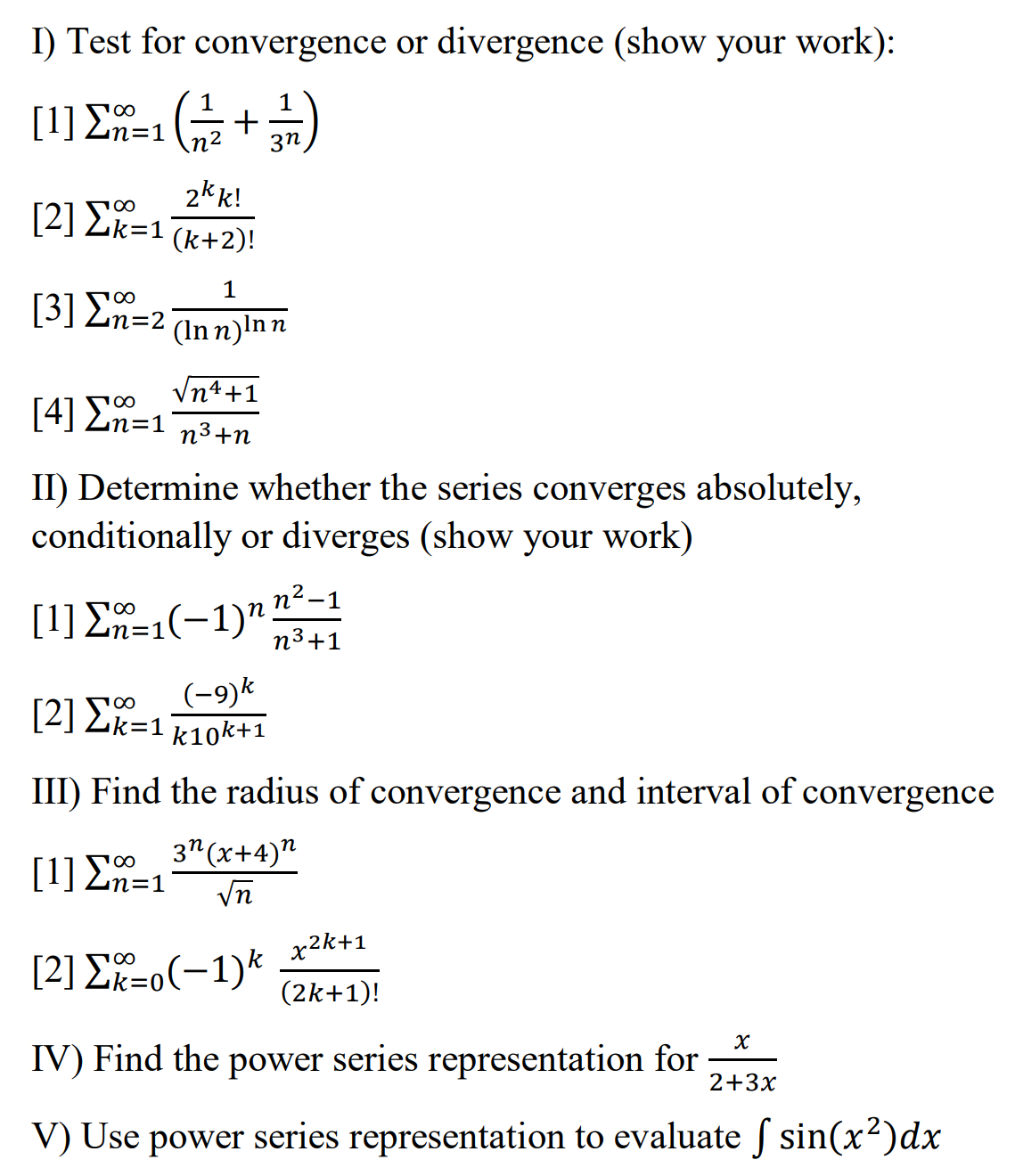### Create an Account

Already have account?

### Forgot Your Password ?

Home / Questions / I) Test for convergence or divergence (show your work):  =1672 + 3) + 2k k!  {k=1 (k...

# I) Test for convergence or divergence (show your work):  =1672 + 3) + 2k k!  {k=1 (k+2)! 1  An-2 (Inn)lnn Vn4+1  An=1 73+1 II) Determine whether the series converges absolutely, conditiona

I) Test for convergence or divergence (show your work):  =1672 + 3) + 2k k!  {k=1 (k+2)! 1  An-2 (Inn)lnn Vn4+1  An=1 73+1 II) Determine whether the series converges absolutely, conditionally or diverges (show your work)  En=1(-1)" n2-1 n3+1  Σ. 100 k=1 (-9) k10k+1 III) Find the radius of convergence and interval of convergence  En=1 3" (x+4)" in  Ek=o(-1)k x2k+1 (2k+1)! х 2+3x IV) Find the power series representation for V) Use power series representation to evaluate S sin(x2)dxApr 14 2021 View more View Less

#### Answer (Solved)Subscribe To Get Solution